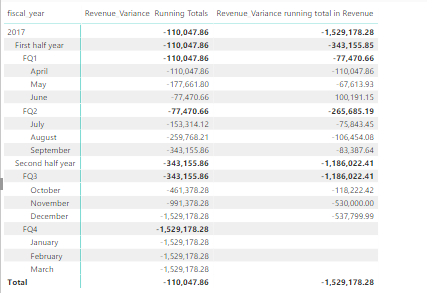cancel
Showing results for
Did you mean:Frequent Visitor

## Rolling totals

I have two DAX queries that I am not sure how to combine to get the outcome that I need. I want my revenue running totals to roll up to Quarter, half year and year like in my second column (in the image below) but also have the cummulated change Month over Month like my first column. Thank you in advance

```Revenue_Variance  Running Totals =
CALCULATE(
'fact_financials'[Revenue_Variance],
FILTER(
CALCULATETABLE(
SUMMARIZE(
'dim_date',
'dim_date'[fiscal_month_number],
'dim_date'[month_name],
'dim_date'[fiscal_quarter],
'dim_date'[fiscal_half_year],
'dim_date'[fiscal_year]
),
ALLSELECTED('dim_date')
),
ISONORAFTER(
'dim_date'[fiscal_year], MIN('dim_date'[fiscal_year]),DESC,
'dim_date'[fiscal_half_year], MIN('dim_date'[fiscal_half_year]),DESC,
'dim_date'[fiscal_quarter], MIN('dim_date'[fiscal_quarter]), DESC,
'dim_date'[fiscal_month_number], MIN('dim_date'[fiscal_month_number]), DESC,
'dim_date'[month_name], MIN('dim_date'[month_name]), DESC
)
)
)```
```Revenue_Variance running total in Revenue =
CALCULATE(
'fact_financials'[Revenue_Variance],
FILTER(
ALLSELECTED('fact_financials'[Revenue]),
ISONORAFTER('fact_financials'[Revenue], MAX('fact_financials'[Revenue]), DESC)
)
)```Microsoft

Hi @lmundia,

Could you post your table structures with just some sample/mock data, and the expected result against the data? So that it will be easier for us to do some tests on it, to see if we can find a solution for you. It's better that you can just share a sample pbix file. You can upload it to OneDrive or Dropbox and post the link here. Do mask sensitive data before uploading.Regards